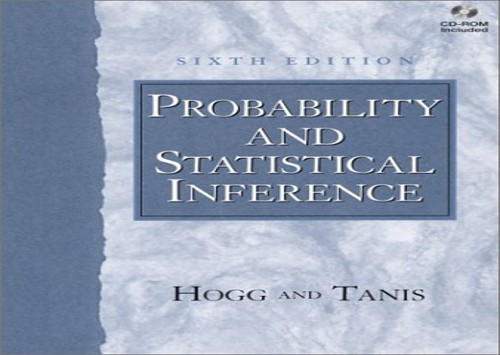# Probability and Statistical Inference by Robert V. Hogg

Out of Stock
\$20.99
inc. GST
This introduction to the mathematics of probability and statistics for students with a background in calculus uses numerous applications to help explain the concepts. This edition contains new features, such as more emphasis on model checking and greater emphasis on confidence intervals.
Only 0 left

## Probability and Statistical Inference Summary

### Probability and Statistical Inference: United States Edition by Robert V. Hogg

For a one/two-semester, junior/senior-level course in Math Stat and/or calculus based Probability and Statistics.

This applied introduction to the mathematics of probability and statistics for students with a background in calculus uses numerous examples, real data based applications, and computer driven exercises to help explain and motivate the concepts.

### Why buy from World of BooksOur excellent value books literally don't cost the earthFree delivery in AustraliaEvery used book bought is one saved from landfill

1. Empirical and Probability Distributions.

Basic Concepts. The Mean, Variance, and Standard Deviation. Continuous-Type Data. Exploratory Data Analysis. Graphical Comparisons of Data Sets. Time Sequences. Probability Density and Mass Functions.

2. Probability.

Properties of Probability. Methods of Enumeration. Conditional Probability. Independent Events. Bayes' Theorem.

3. Discrete Distributions.

Random Variables of the Discrete Type. Mathematical Expectation. Bernoulli Trials and the Binomial Distribution. The Moment-Generating Function. The Poisson Distribution.

4. Continuous Distributions.

Random Variables of the Continuous Type. The Uniform and Exponential Distributions. The Gamma and Chi-Square Distributions. The Normal Distribution. Distributions of Functions of a Random Variable. Mixed Distributions and Censoring.

5. Multivariable Distributions.

Distributions of Two Random Variables. The Correlation Coefficient. Conditional Distributions. The Bivariate Normal Distribution. Transformations of Random Variables. Order Statistics.

6. Sampling Distribution Theory.

Independent Random Variables. Distributions of Sums of Independent Random Variables. Random Functions Associated with Normal Distributions. The Central Limit Theorem. Approximations for Discrete Distributions. The t and F Distributions. Limiting Moment-Generating Functions. Chebyshev's Inequality and Convergence in Probability.

Importance of Understanding Variability.
7. Estimation.

Point Estimation. Confidence Intervals for Means. Confidence Intervals for Difference of Two Means. Confidence Intervals for Variances. Confidence Intervals for Proportions. Sample Size. Distribution-Free Confidence Intervals for Percentiles. A Simple Regression Problem. More Regression.

8. Tests of Statistical Hypotheses.

Tests about Proportions. Tests about One Mean and One Variance. Tests of the Equality of Two Normal Distributions. Chi-Square Goodness of Fit Test. Contingency Tables. Tests of the Equality of Several Means. Two-Factor Analysis of Variance. Tests Concerning Regression and Correlation. The Wilcoxon Tests. Kolmogorov-Smirnov Goodness of Fit Test. Resampling Methods. Run Test and Test for Randomness.

9. Theory of Statistical Inference.

Sufficient Statistics. Power of a Statistical Test. Best Critical Regions. Likelihood Ratio Tests. Bayesian Estimation. Asymptotic Distributions of Maximum Likelihood Estimators.

10. Quality Improvement through Statistical Methods.

Statistical Quality Control. General Factorial and 2k Factorial Designs. More on Design of Experiments.

Epilogue.Appendix A. Review of Selected Mathematical Techniques.

Algebra of Sets. Mathematical Tools for the Hypergeometric Distribution. Limits. Infinite Series. Integration. Multivariate Calculus.

Appendix B. References.Appendix C. Tables.Appendix D. Answers to Odd-Numbered Exercises.Index.

GOR003021834
Probability and Statistical Inference: United States Edition by Robert V. Hogg
Robert V. Hogg
Used - Very Good
Hardback
Pearson Education (US)
2000-07-25
704
0130272949
9780130272942
N/A
Book picture is for illustrative purposes only, actual binding, cover or edition may vary.
This is a used book - there is no escaping the fact it has been read by someone else and it will show signs of wear and previous use. Overall we expect it to be in very good condition, but if you are not entirely satisfied please get in touch with us.